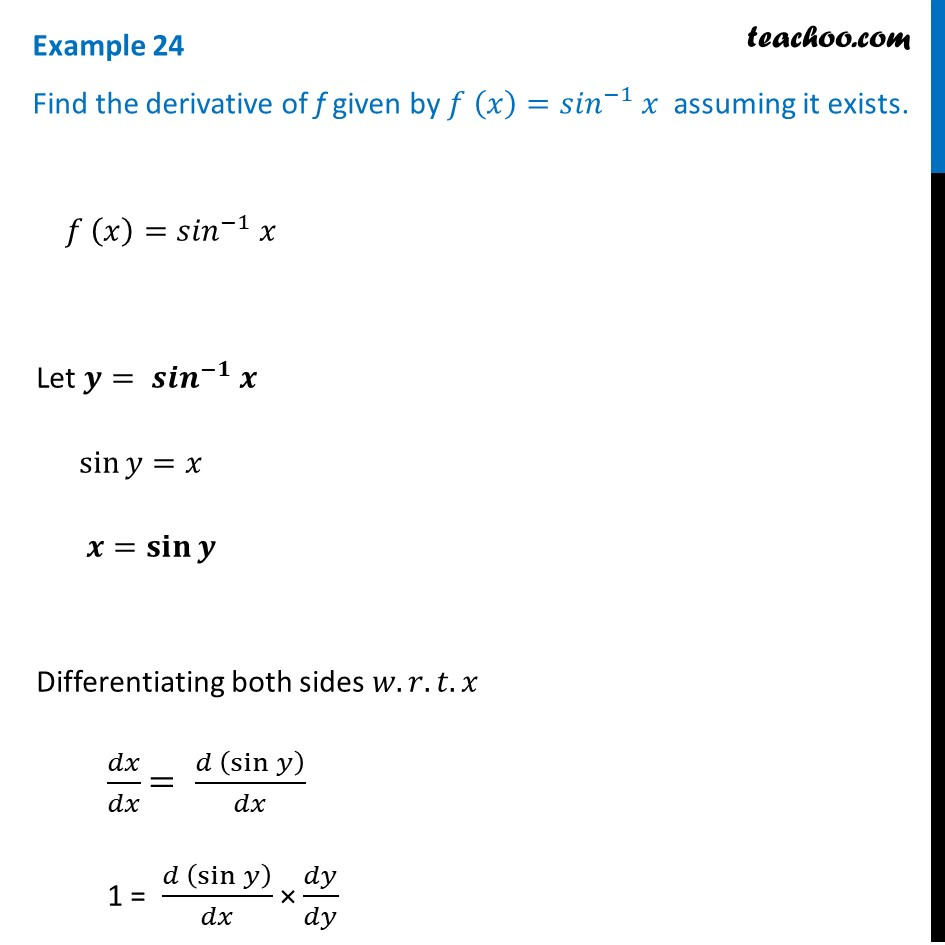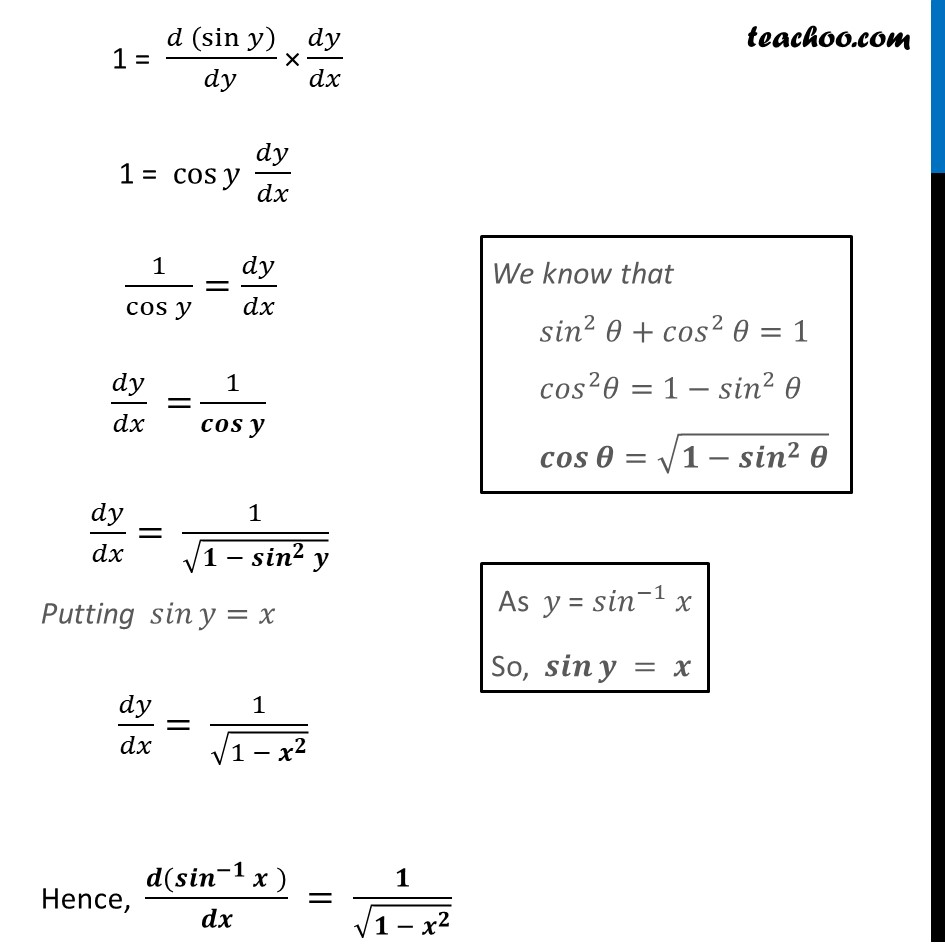Examples

Chapter 5 Class 12 Continuity and Differentiability
Serial order wiseLearn in your speed, with individual attention - Teachoo Maths 1-on-1 Class

### Transcript

Example 24 Find the derivative of f given by 𝑓 (𝑥)=〖𝑠𝑖𝑛〗^(−1) 𝑥 assuming it exists. 𝑓 (𝑥)=〖𝑠𝑖𝑛〗^(−1) 𝑥 Let 𝒚= 〖𝒔𝒊𝒏〗^(−𝟏) 𝒙 sin⁡〖𝑦=𝑥〗 𝒙=𝐬𝐢𝐧⁡〖𝒚 〗 Differentiating both sides 𝑤.𝑟.𝑡.𝑥 𝑑𝑥/𝑑𝑥 = (𝑑 (sin⁡𝑦 ))/𝑑𝑥 1 = (𝑑 (sin⁡𝑦 ))/𝑑𝑥 × 𝑑𝑦/𝑑𝑦 1 = (𝑑 (sin⁡𝑦 ))/𝑑𝑦 × 𝑑𝑦/𝑑𝑥 1 = cos⁡𝑦 𝑑𝑦/𝑑𝑥 1/cos⁡𝑦 =𝑑𝑦/𝑑𝑥 𝑑𝑦/𝑑𝑥 = 1/𝒄𝒐𝒔⁡𝒚 𝑑𝑦/𝑑𝑥= 1/√(𝟏 − 〖𝒔𝒊𝒏〗^𝟐 𝒚) Putting 𝑠𝑖𝑛⁡〖𝑦=𝑥〗 𝑑𝑦/𝑑𝑥= 1/√(1 − 𝒙^𝟐 ) Hence, (𝒅(〖𝒔𝒊𝒏〗^(−𝟏) 𝒙" " ))/𝒅𝒙 = 𝟏/√(𝟏 − 𝒙^𝟐 ) "We know that" 〖𝑠𝑖𝑛〗^2 𝜃+〖𝑐𝑜𝑠〗^2 𝜃=1 〖𝑐𝑜𝑠〗^2 𝜃=1−〖𝑠𝑖𝑛〗^2 𝜃 𝒄𝒐𝒔⁡𝜽=√(𝟏−〖𝒔𝒊𝒏〗^𝟐 𝜽) " " As 𝑦 = 〖𝑠𝑖𝑛〗^(−1) 𝑥 So, 𝒔𝒊𝒏⁡𝒚 = 𝒙